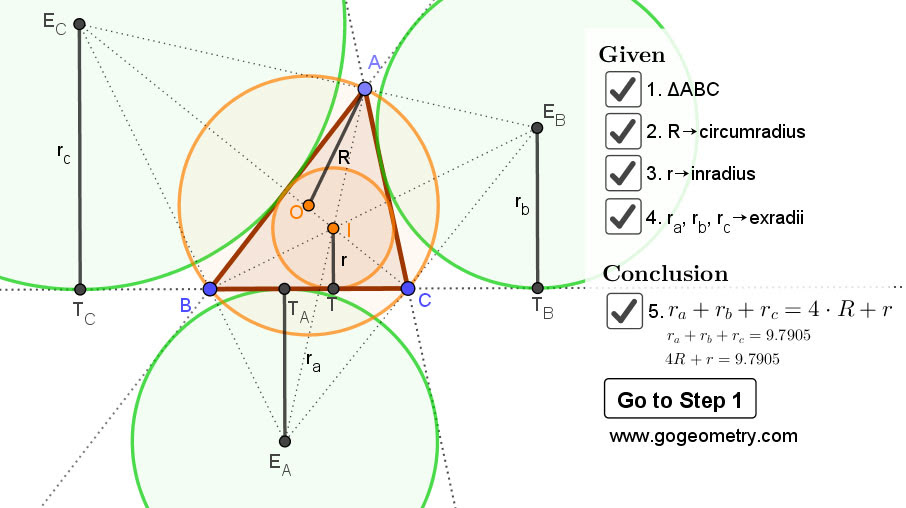## Sunday, April 5, 2020

Interactive step-by-step animation using GeoGebra. Post your solution in the comment box below.
Level: Mathematics Education, High School, Honors Geometry, College.

Details: Click on the figure below.1.https://photos.app.goo.gl/BAb6FnZVGoRYxj5j6

Let 2p= perimeter of triangle ABC
Draw diameter KL of circle O (see sketch)
We have KL=MK+ML=2R….(1)
Note that M is the midpoint of TTa and TcTb ( see sketch)
In trapezoid EcEbTbTc we have MK=1/2(Rc+Rb)…(2)
In trapezoid ITEaTa we have ML=1/2(Ra-r)…. 3)
Combine (1), (2) and (3) we have 2R=1/2(Rc+Rb)+1/2(Ra-r)
And 4R+r= Ra+Rb+Rc

2.Draw NOMP perpendicular to BC (N,M on O). M midpoint of TcTb => MN middle line of trapez
MN = (Rc+Rb)/2 (1)
BTa=TC => MTa=MT. Draw NS perpnd to EaTa From congruece of tr
MP+r=Ra-MP (2)
From (1) and (2) Ra+Rb+Rc=4R+r